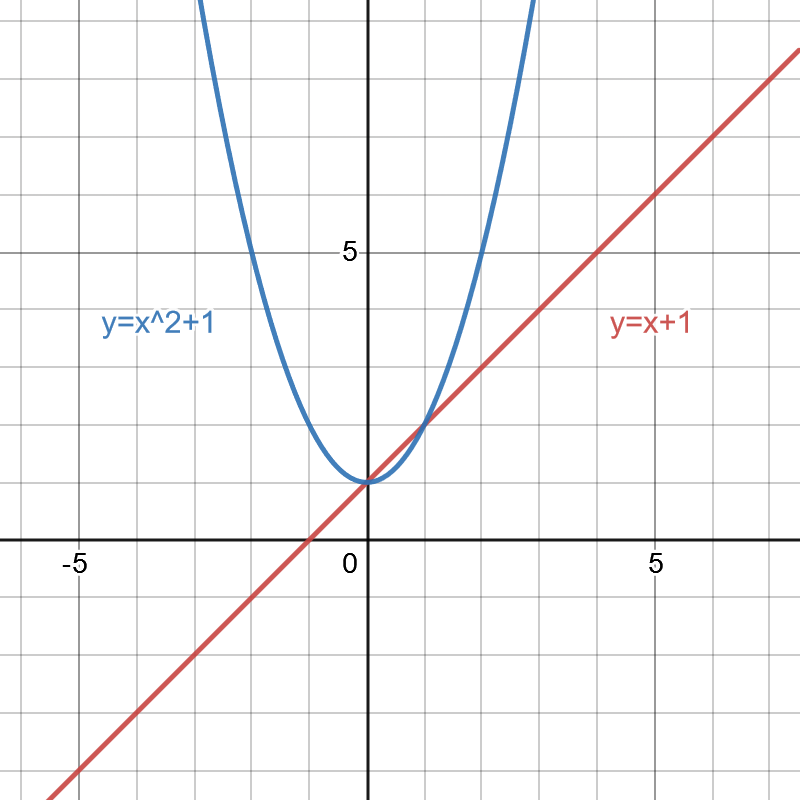Now that we have learnt about system of linear equations and how to solve it, let’s move on to non-linear system of equations.

Non-linear system of equations

Non-linear equations are equations that cannot be linearly plotted, and hence cannot be plotted on a graph as a straight line. The highest degree of the variables inside a non-linear equation is higher than 1. An example of a non-linear equation is$y=x^2+1$

A system of non-linear equations refers to a system of equations in which a non-linear equation is present, though not all equations inside the system needs to be non-linear. It also differs from a linear system as it can also have more than one solution. The following is an example of a non-linear system of equations with 2 equations with variables$x$ and$y$.$y=x+1$$y=x^2+1$

The concept of solving a non-linear system of equations is similar to that of a linear system of equation, as we are trying to find the point of intersection for all of the graphs. Using the above system as an example, by plotting it in a graph,And from the 2 points of intersection, we can deduce the 2 solutions, which are$x=0$$y=1$

and$x=1$$y=2$

We are also able to use substitution to obtain our results. Using the previous system as an example, we can use the equation$x=y-1$

and substitute it into$y=x^2+1$$y=(y-1)^2+1$$y=y^2-2y+1+1$$0=y^2-3y+2$

By completing the root, we will get$0=(y-2)(y-1)$

### Real World Examples!

Did you know? One of the best known and most used case of non-linear system of equations is calculating alternating current power-flow model in an electric power system, as they use non-linear equations to calculate power for each transmission line.

Solving this system gives the flow of active power in an electrical system. It is the same flow of power that powers your home and keeps the computer charging, and is very important for analysing power grids. The system of equations is expressed as:$\begin{bmatrix}\Delta \theta \\\Delta |V|\end{bmatrix}=-J^{-1}\begin{bmatrix}\Delta P\\\Delta Q\end{bmatrix}$

				
%use s2
//f1:y=x+1
val f1:BivariateRealFunction = object : AbstractBivariateRealFunction() {
//Using AbstractBivariateRealFunction as we are only using 2 variables
override fun evaluate(x: Double, y: Double): Double {
//if the values are correct, it returns 0
return x + 1 - y
}}

//f2:y=x^2+1
val f2:BivariateRealFunction = object : AbstractBivariateRealFunction() {
//Using AbstractBivariateRealFunction as we are only using 2 variables
override fun evaluate(x: Double, y: Double): Double {
//if the values are correct, it returns 0
return x*x + 1 - y
}}

println(f1.evaluate(1.0,2.0))
println(f2.evaluate(1.0,2.0))



As systems of nonlinear equations becomes more complex, it will be harder to obtain solutions by substitutions or graphing. In the next chapter, we will be looking at the theory behind Newton’s method, as well as how to implement it in NMdev, to be able to find solutions to more complex non-linear systems.